C L DUBEY – EXERCISE – 02 : Simpson’s Rule |
Miscelleneous

# C L DUBEY – EXERCISE – 02 : Simpson’s Rule

###### Draft (m)
6.0 5.0 4.0 3.0 2.0
###### TPC
22.45 22.04 21.53 20.91 19.68

Given,

###### Moment
6.0 22.45 (100)⨯22.45/1.025 1 22.45x 4h 89.8xh
5.0 22.04 22.04x 4 88.16x 3h 264.48xh
4.0 21.53 21.53x 2 43.06x 2h 86.12xh
3.0 20.91 20.91x 4 83.64x 1h 83.64xh
2.0 19.68 19.68x 1 19.68x 0h 0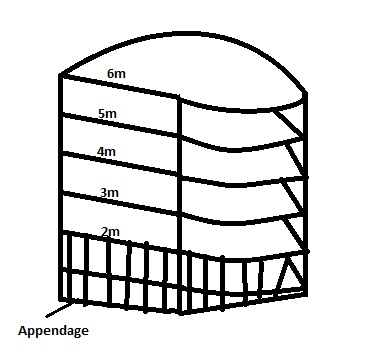Sum of product of volume = 256.99x

Volume = 1/3 ⨯ 256.99 ⨯ 100/1.025
= 8357.398m3

Sum of product of moment = 524.04 ⨯ 1/3 ⨯ 100/1.025 ⨯ 1
= 17041.95m4

Total volume including appendage =?
Given displacement of appendage = 3280 tonnes at draft 2m
We know displacement = ( v/w volume X  density of water displaced)
Volume of appendage = 3280/1.025
= 3200m3

Total volume including appendage = (8357.398 + 3200) m3
= 11557.398 m3

COG of the structure without appendage
= volume/Moment
= 2.039m

COG from 2.0 mtr draft = 2.039 m
Now total moment = moment of appendage + moment of structure
11846.33 ⨯  X   = (3280 ⨯ 2) + (8566.33 ⨯ 4.039)

###### Product
1.2 1 1.2
9.6 4 38.4
13.2 2 26.4
15.0 4 60.0
15.3 2 30.6
15.6 4 62.4
15.6 2 31.2
14.7 4 58.8
12.9 2 25.8
9.0 4 36.0
0.0 1 0.0
370.8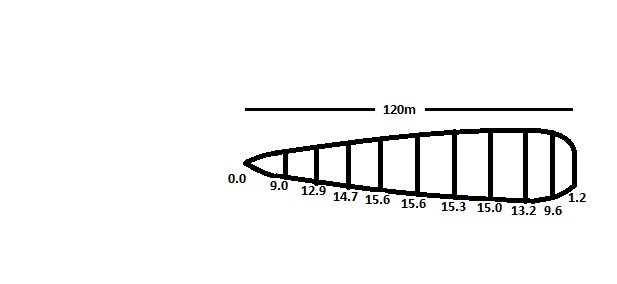Water plane Area = ( H x SOP )/ 3
= 1483.2m2

We know that – TPC = A/100 ⨯ density
= 15.2 tonnes

FWA =    W/40 ⨯ TPC
=     6690/40 ⨯ 15.2

###### Product
0.1 0.5 0.05
7.5 2 15
10 1.5 15
12 4 48
12.3 2 24.6
11.4 4 45.6
8 1.5 12
5.2 2 10.4
1.0 0.5 0.5
SOP = 171.15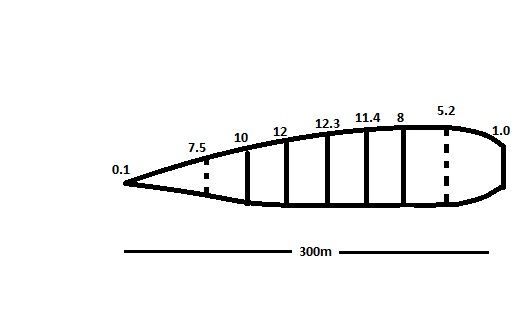Area = 50/3  ⨯ 171.15
= 2852.5m2

###### Product of moment
800 1 800 0h 0
760 4 3040 h 3040h
700 2 1400 2h 2800
600 4 2400 3h 7200h
450 1.5 675 4h 2700h
180 2 360 4.5h 1620h
10 0.5 05 5h 25h
SOP = 8680   SOM = 17385h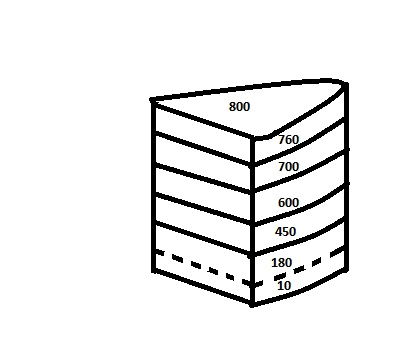Volume = 8680/3
= 2893.33

###### Displacement = 2965.67 tonnes

COG from Top = 2.00288m
COG from bottom = 2.99m

###### Q5. A wall-sided vessel of constant water-plane area of length 105m has the following equally spaced half-ordinates of water plane: 1, 7, 3.4, 5.6, 6.7, 6.7, 5.6, 3.4 and 1.7 mtrs. When floating at an even keel draft of 4.5m, an empty compartment with transverse end bulkheads at the 6.7m ordinates is bilged. Calculate the end draft at which she would settle.

Solution –  Will be Uploaded Soon.

###### The after bulkhead is 65m forward of the centre of floatation. Calculate the change of trim if the is filled with fuel oil density 0.95, MCTC 200 tm.

Solution –  Will be Uploaded Soon.

###### Product of moment
0 5850 1 5850     0h    0h
1 5885 4 23540 1h 23540h
2 5900 2 11800 2h 23600h
3 5915 4 23660 3h 70980h
4 5943 2 11886 4h 47544h
5 5975 4 23900 5h 119500h
6 5995 1 5995 6h 35970h
SOP = 106631   SOM = 321134h
###### KB = 3.012m

Volume = 3554.667
W = 36432.258

TPC = A/100 ⨯ density of water
= 61.448

We can calculate – FWA = W/40 ⨯ TPC

###### moment
19.2 1 19.2 0h 0
18.0 4 72.0 3h 216
17.1 2 34.2 6h 205.2
16.2 4 64.8 9h 583.2
14.4 1 14.4 12h 172.8
SOP = 204.6   SOM = 1179.2h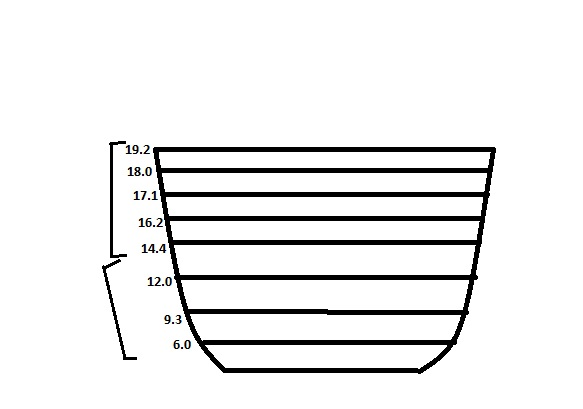Moment = 3/3 ⨯ 1179.2
= 1179.2

Area = 3/3 ⨯ 204.6
= 204.6

###### Moment
14.4 1 14.4 12h 172.8h
12.0 3 36.0 15h 540h
9.3 3 27.9 18h 502.2h
6.0 1 6.0 21h 126h
SOP=84.3   SOM = 1341h

Moment = (3 ⨯ 3/8) ⨯ 1341
= 1508.625

Area = 9/8 ⨯ 84.3
= 94.83

Total moment = 2685.825
Area = 299.43

Therefore,
Geometric Centre = ( Total moment / Area)

###### Moment
0.4 0.5 0.2 0h 0h
4.4 2 8.8 0.5h 4.4h
8.8 1.5 13.2 h 13.2h
11.0 4 44.0 3h 8.8h
11.6 2 23.2 3h 69.6h
11.8 24 47.2 4h 181.8h
11.8 1.5 23.6 5h 118h
11.6 4 46.4 6h 278h
9.6 1.5 14.4 7h 100.8h
7.0 2 14.0 7.5h 105h
0.4 0.5 0.2 8h 1.6h
SOP=235.2   SOM=967.8h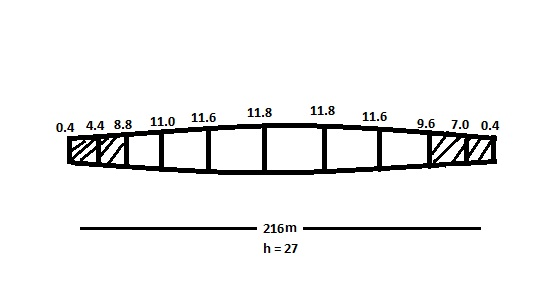Area = 2 ⨯ 27/3 ⨯235.2
= 4233.6m2

COG from one end = 111.099

###### Solution –

Using Simpson’s 3 rules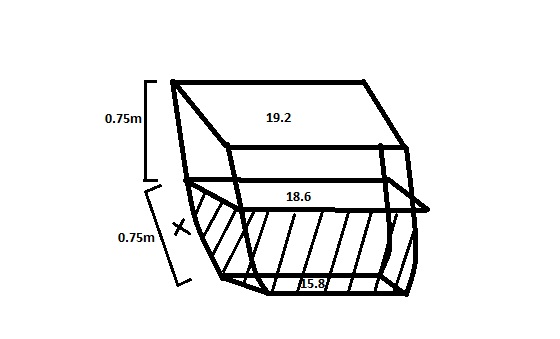Volume = 0.75/1 [5 ⨯ 158 + 8 ⨯ 186 – 196]
= 130.375m3

###### Weight = 131.94 tonnes

For moment = 0.75/12 [3 ⨯ 158 + 8 ⨯ 186 – 196]
= 50.203m4

###### Solution –

Original even Keel draft = 5.2m

###### Product area
0 1   0
5 4 20
9 2 18
10 4 40
9 2 18
5 4 20
0 1 0
SOP = 116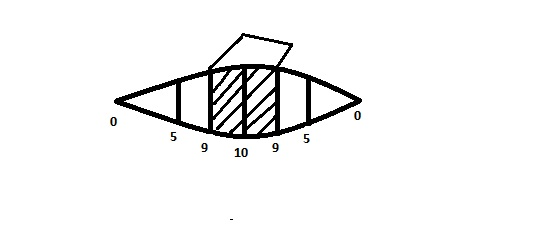Water Plane area = 25/3 ⨯ 116
= 966.67 ⨯ 2
= 1933.33m2

TPC = 1933.33/100 ⨯ 1.025
= 19.816

Volume between ordinate of 9m & 9m =?
Area between 9m & 9m = 25/12 ⨯ [45 + 80 – 9] ⨯2 ⨯ 2
= 966.67m2

Volume between 9m & 9m = 966.67m2
Weight of water = 990.83 tonnes
Sinkage = W/TPC
= 0.5m

Therefore, final draft = 5.7m = (5.2 + 0.5)
Thrust on tank top = (Thrust due to draft – Thrust due to tank water)

Thrust = Depth ⨯ Density ⨯ Area
= (5.7 ⨯ 1.025 ⨯ 977.07) – (1 ⨯ 1.025 ⨯ 966.07)
= 5647.769 – 990.83

###### POM
0.0 0.5 0   0 0
2.0 2 4 6 24
3.3 1.5 4.95 12 59.4
4.5 4 18 24 432
4.8 2 9.6 36 345.6
4.5 4 18 48 864
3.6 2 7.2 60 432
2.7 4 10.8 72 777.6
1.5 1 1.5 84 126
74.05   3060.6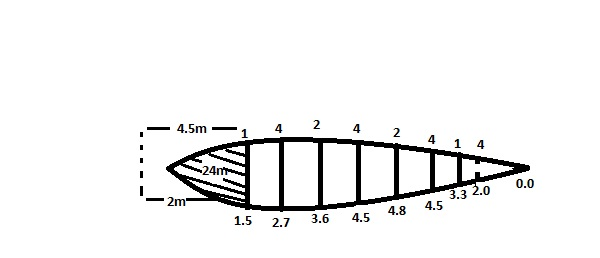Area = (2 ⨯ 12/3) ⨯ 74.05
= 592.4m2

###### Total area = 616.4 m2

COG from aft = 41.33m
COG = (592.4 ⨯ 41.33) + (24 ⨯ 86.5)/616.4

###### Solution –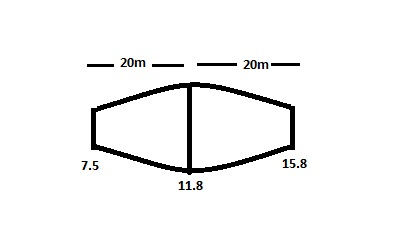Area between first two ordinate
= 2 ⨯ (20/12) ⨯ [5 ⨯ 7.5 + 8 ⨯ 11.8 – 15.8]
= 387m2

Area between last two ordinate
= 2 ⨯ (20/12) ⨯ [5 ⨯ 15.8 + 8 ⨯ 11.8 – 7.5]
= 553m2

Moment between first two ordinate
= 2 ⨯ (20 ⨯ 20/24) [3 ⨯ 7.5 + 10 ⨯ 11.8 – 15.8]
= 4156.67 tm

Moment between first last two ordinate from aft
= 2 ⨯ (20 ⨯ 20/24)  [3 ⨯ 15.8 + 10 ⨯ 11.8 – 7.5]
= 5263.335 tm

###### Product of volume
8.2 8.2x 1 8.2x
16.5 16.5x 4 66x
18.7 18.7x 2 37.4x
19.4 19.4x 4 77.6x
20.0 20.0x 2 40.0x
20.5 20.5x 4 82.0x
21.1 21.1x 1 21.1x
332.2x

TPC = (A/100) x density
A = TPC ⨯ (100/density)
X = 100/1.025

V/W volume = (1.2/3) ⨯ 332.3 ⨯ 100/1.025
= 12967.804

###### Displacement = (v/w volume ⨯ density) = 13291.9 tonnes